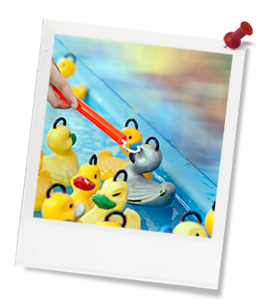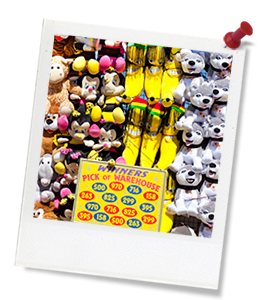## Activity IntroductionQuick summary: In this lesson, students will plan a budget for the running of their sideshow game at a real or imagined student fair or fete, and then record and calculate revenue, cost and profit (or loss) from the (real or imagined) takings from operating the game.

Calculating the ‘break-even’ point for their game will be a critical practical implication for the potential use of their game in a student-led fundraising fete or fair, as a possible (optional) post-unit activity.

This lesson sequence will require 2 standard classroom periods of 45-50 minutes to complete.

Learning intentions:

• Students will understand basic business budgeting concepts, including fixed costs (expenses), variable costs (expenses), total costs (expenses), revenue, profit, loss and break-even
• Students will use mathematical reasoning to ensure a reasonable and viable price for their Sideshow Game and learn how to use financial estimates within a simple budget
• Students will use a pre-prepared spreadsheet template to record their budget information and generate a graphical representation of both costs and revenues to predict a break-even point in the sale of their Sideshow Game ‘product’.

21st century skills:

### Australian Curriculum Mapping

Content descriptions:

Years 5 to 8 Mathematics:

• Create simple financial plans (ACMNA106)
• Solve problems involving profit and loss, with and without digital technologies (ACMNA189)

Syllabus outcomes: MA3‑1WM, MA3‑2WM, MA3‑3WM, MA3‑5NA

Relevant parts of Year 5 to 8 achievement standards:

By the end of Year 5, students can check the reasonableness of their calculations using estimation. Students use mathematical modelling to solve financial and other practical problems, formulating and solving problems, choosing arithmetic operations and interpreting results in terms of the situation. They interpret and compare data represented in line graphs. Students conduct repeated chance experiments, list the possible outcomes, estimate likelihoods and make comparisons between those with and without equally likely outcomes.

Topic: STEAM/STEAM, Sustainability.

This lesson is part of the wider unit of work: Sustainable STEM Sideshow.

Time required: 90 mins (approx. two standard lessons).

Level of teacher scaffolding: Medium – As a teacher, you will need to explain basic business budgeting concepts including fixed costs (expenses), variable costs (expenses), total costs (expenses), revenue, profit, loss and break-even.

You’ll also need to help students estimate each of these items and use these financial estimates within a budget, including the basic operation of Excel spreadsheets OR manually plotting graph lines for each of the above items.

Resources required:

• Electronic whiteboard or data projector, for demonstrating and discussing lesson content
• Excel spreadsheet template document, distributed to each student (or student team): My STEM Sideshow P&L Plan
• Student Worksheet for Lesson 4: Profit and Loss (Financial Mathematics) (one per student)

Keywords: fixed costs (expenses), variable costs (expenses), total costs (expenses), revenue, profit, loss, break-even, spreadsheet, cost curve, revenue curve, profit curve.

Cool Australia’s curriculum team continually reviews and refines our resources to be in line with changes to the Australian Curriculum.

##Teacher Preparation

Learning intentions: Students will...

• ... understand basic business budgeting concepts, including fixed costs (expenses), variable costs (expenses), total costs (expenses), revenue, profit, loss and break-even
• ... use mathematical reasoning to ensure a reasonable and viable price for their Sideshow Game and learn how to use financial estimates within a simple budget
• ... use a pre-prepared spreadsheet template to record their budget information and generate a graphical representation of both costs and revenues to predict a break-even point in the sale of their Sideshow Game ‘product’.

Success criteria: Students can…

• ... identify each of the revenue, fixed cost and variable cost items for the building and proposed running of their Sustainable STEM Sideshow Game
• ... calculate each of Total Cost and Profit (or Loss) from the above items
• ... plan a profit and loss budget (‘P&L’) for the running of their Sustainable STEM Sideshow Game at a real or imag
...

## Thought starter: 'Remind people that profit is the difference between revenue and expense. This makes you look smart.' - Scott Adams

Let’s think about what you did back in Lesson 2 when we looked at the probability of a player winning your Sustainable STEM Sideshow Game.

Grab your Lesson 2 Student Worksheet, because you’ll need some of your working out to get going on our lesson today.

•  Write down the event frequency of recording a win in your game as a ratio, in the same way as you did in your Lesson 2 Student Worksheet::Total wins Total turns

•  Record the expected cost of each prize you plan to award game players when they win. If the prize prices vary, you will need to work out an average prize cost (total sum of all the prize costs to you, divided by the number of prizes you have bought).
Prize cost: \$.

•  Make a reasonable estimate of the number of turns you think you will be able to manage during the running of your game.

•  Choose a time peri

...